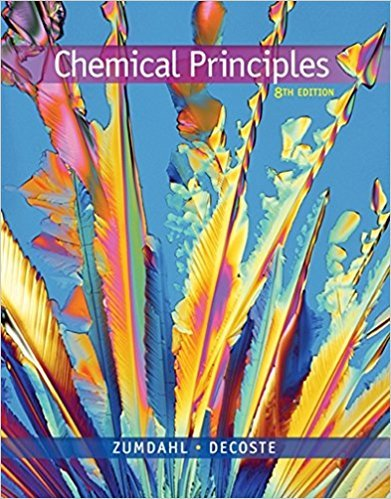×
×

# Answer: A 30.0-mL sample of an unknown strong base isISBN: 9781305581982 176

## Solution for problem 4.66 Chapter 4

Chemical Principles | 8th Edition

• Textbook Solutions
• 2901 Step-by-step solutions solved by professors and subject experts
• Get 24/7 help from StudySoup virtual teaching assistantsChemical Principles | 8th Edition

4 5 1 281 Reviews
10
4
Problem 4.66

A 30.0-mL sample of an unknown strong base is neutralized after the addition of 12.0 mL of a 0.150 M HNO3 solution. If the unknown base concentration is 0.0300 M, give some possible identities for the unknown base.

Step-by-Step Solution:
Step 1 of 3
Step 2 of 3

Step 3 of 3

##### ISBN: 9781305581982

Chemical Principles was written by and is associated to the ISBN: 9781305581982. This full solution covers the following key subjects: . This expansive textbook survival guide covers 20 chapters, and 2754 solutions. The answer to “A 30.0-mL sample of an unknown strong base is neutralized after the addition of 12.0 mL of a 0.150 M HNO3 solution. If the unknown base concentration is 0.0300 M, give some possible identities for the unknown base.” is broken down into a number of easy to follow steps, and 38 words. The full step-by-step solution to problem: 4.66 from chapter: 4 was answered by , our top Chemistry solution expert on 12/23/17, 04:53PM. This textbook survival guide was created for the textbook: Chemical Principles, edition: 8. Since the solution to 4.66 from 4 chapter was answered, more than 237 students have viewed the full step-by-step answer.

Unlock Textbook Solution